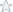# Search our library of math homework solutions using Google

## TECHBUS Business Math Midterm and Final Exam Solutions

Topic: College Algebra
Details: Questions [ Print this page } Course: TECHBUS Business Math Unit: Midterm Exam Answer the following questions below: Page 1 of 16. For problems #1 through #3, find the weekly earnings. All hours over 40 hours are paid at timeand- a-half. 1) \$4.75 p...
Rating:5.00 of 5 Stars based on 5 review(s)  [Download Solutions]

## VLA Math Algebra 1 - Various Units - See files for details

Topic: Basic Algebra
Details: Course: VLA Math Algebra 1_1 Unit: Proportions, Percents, and Statistics Unit: Functions and Relations A LOT OF OTHER UNITS AS WELL. SEE FILES FOR DETAILS!
Tags: Algebra Homework solutions
Rating:5.00 of 5 Stars based on 1 review(s)  [Download Solutions]

## Mixed Math Algebra and Calculus Problems

Topic: General Coursework
Details: 1. Find the domain of the function f(x) = . 1) ______ (A) D = (- , 2) (2, ) (B) D = (- , -2) (-2, ) (C) D = (2, ) (D) D = (-2, ) (E) none of the above 2. A cell phone company offers a plan of \$39 per month for 400 minutes. Each additiona...
Tags: Algebra Homework solutions
Rating:4.40 of 5 Stars based on 30 review(s)  [Download Solutions]

## Section 3.5 Written Homework

Topic: Inverse Functions Written Homework
Details: Section 3.5: Inverse Functions Written Homework Remember: Any submissions to my email after 11:59pm will NOT be accepted and will result in a grade of a 0. Homework must be presented in an orderly manner with the problems in order, running in a single c...
Tags: algebra, section, section 3.5, written, homework, inverse, functions
Rating:5.00 of 5 Stars based on 1 review(s)  [Download Solutions]

## MATH 107 6383 - UMUC - Quiz 1

Topic: Math107 Quiz 1
Details: MATH 107 6383, Fall 2014 UMUC Quiz 1: Show your work on all the problems. Submit the completed quiz in the assignment folder. Total Points: 100 Due: August 31, 2014 1. Find the distance between -7 and 3 on the number line. ...
Tags: Math107, MATH 107, quiz, UMUC, College, Algebra
Rating:4.90 of 5 Stars based on 4 review(s)  [Download Solutions]

## Midterm - MATH 107 - 6383 Fall 2014

Topic: MATH 107 UMUC Midterm Exam
Details: MATH 107 6383, Fall, 2014 UMUC Midterm Exam Show your work on all the problems. Submit your completed exam in the assignment folder. Total Points: 100 Due: September 21 1. a. Find the midpoint of the segment with endpoints (5...
Tags: Math107, MATH 107, midterm, exam, UMUC, College, Algebra
Rating:5.00 of 5 Stars based on 3 review(s)  [Download Solutions]

## MATH 107 UMUC Final Examination

Topic: College Algebra MATH 107 UMUC Final Exam
Details: College Algebra MATH 107 Fall, 2014, V1.3 Page 1 of 11 MATH 107 FINAL EXAMINATION This is an open-book exam. You may refer to your text and other course materials as you work on the exam, and you may use a calculator. You must complete the exam individ...
Tags: Math107, MATH 107, final, exam, UMUC, College, Algebra
Rating:3.00 of 5 Stars based on 20 review(s)  [Download Solutions]

## MA1310 - Week 5 Law of Sines and Cosines

Topic: Law of Sines and Cosines, Heron’s formula, Triangle solving
Details: This lab requires you to: • Use the Law of Sines to solve oblique triangles. • Use the Law of Cosines to solve oblique triangles. • Use Heron’s formula to find the area of a triangle. • Solve applied problems. Answer the following questions to comple...
Tags: MA1310, MA 1310, week5_lab, law of sines, law of cosines, heron's formula
Rating:5.00 of 5 Stars based on 1 review(s)  [Download Solutions]

## MATH 115 Quiz 5 - Trigonometry

Topic: trigonometric equations, triangle solving, vector operations, applications on vectors
Details: MATH 115 Quiz 5 NAME___________________________ Professor: Dr. Izmirli INSTRUCTIONS The quiz is worth 100 points. There are five problems (each worth 20 points). This quiz is open book and open notes. This means you may refer to your textbook, n...
Tags: MATH115, MATH 115, Quiz 5, UMUC, trigonometry
Rating:5.00 of 5 Stars based on 1 review(s)  [Download Solutions]